# AQA A Level Maths: Statistics复习笔记4.3.1 The Normal Distribution

### Properties of Normal Distribution

The binomial distribution is an example of a discrete probability distribution. The normal distribution is an example of a continuous probability distribution.

#### What is a continuous random variable?

• A continuous random variable (often abbreviated to CRV) is a random variable take any value within a range of infinite values
• Continuous random variables usually measure something
• For example, height, weight, time, etc

#### What is a continuous probability distribution?

• A continuous probability distribution is a probability distribution in which the random variable X is continuous
• The probability of X being a particular value is always zero
• P(X = k) = 0 for any value k
• Instead we define the probability density function f(x) for a specific value
•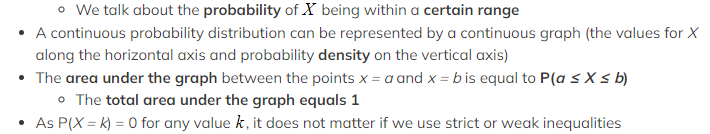P(X ≤ k) = P(X < k) for any value k

#### What is a normal distribution?

• A normal distribution is a continuous probability distribution
• The continuous random variable can follow a normal distribution if:
• The distribution is symmetrical
• The distribution is bell-shaped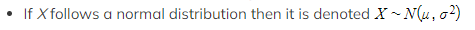• μ is the mean
• σ2 is the variance
• σ  is the standard deviation
• If the mean changes then the graph is translated horizontally
• If the variance changes then the graph is stretched horizontally
• A small variance leads to a tall curve with a narrow centre
• A large variance leads to a short curve with a wide centre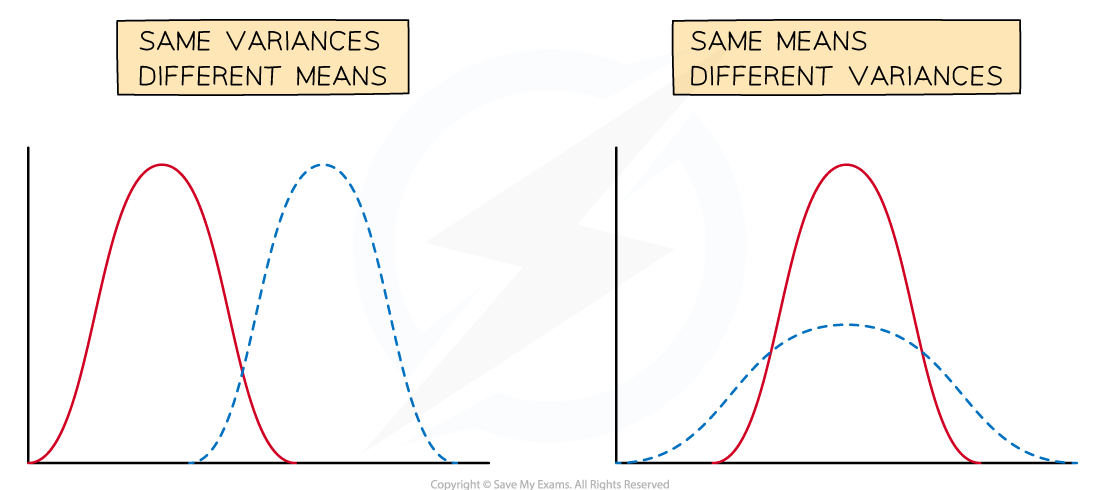#### What are the important properties of a normal distribution?

• The mean is μ
• The variance is σ2
• If you need the standard deviation remember to square root this
• The normal distribution is symmetrical about x = μ
• Mean = Median = Mode = μ
• The normal distribution curve has two points of inflection
• x = μ ±  σ (one standard deviation away from the mean)
• There are the results:
• Approximately two-thirds (68%) of the data lies within one standard deviation of the mean (μ ±  σ)
• Approximately 95% of the data lies within two standard deviations of the mean (μ ± 2σ)
• Nearly all of the data (99.7%) lies within three standard deviations of the mean (μ ± 3σ)
• For any value x a z-score (or z-value) can be calculated which measures how many standard deviations x is away from the mean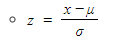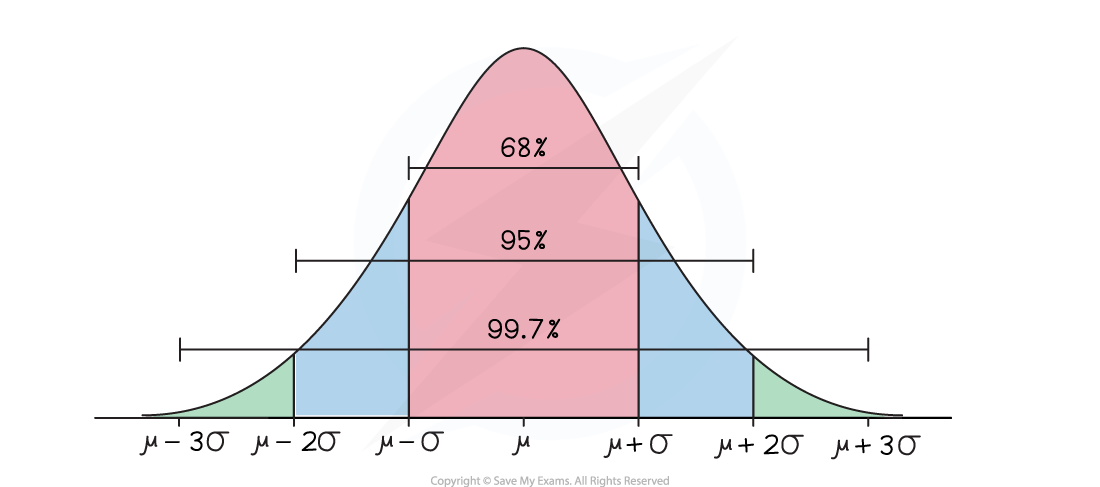### Modelling with Normal Distribution

#### What can be modelled using a normal distribution?

• A lot of real-life continuous variables can be modelled by a normal distribution provided that the population is large enough and that the variable is symmetrical with one mode
• For a normal distribution X can take any real value, however values far from the mean (more than 4 standard deviations away from the mean) have a probability density of practically zero
• This fact allows us to model variables that are not defined for all real values such as height and weight

#### What can not be modelled using a normal distribution?

• Variables which have more than one mode or no mode
• For example, the number given by a random number generator
• Variables which are not symmetrical
• For example, how long a human lives for

#### Worked Example

The random variable S represents the speeds (mph) of a certain species of cheetahs when they run. The variable is modelled using N(40, 100).

(a)Write down the mean and standard deviation of the running speeds of cheetahs.
(b)State the two assumptions that have been made in order to use this model.
(a)Write down the mean and standard deviation of the running speeds of cheetahs.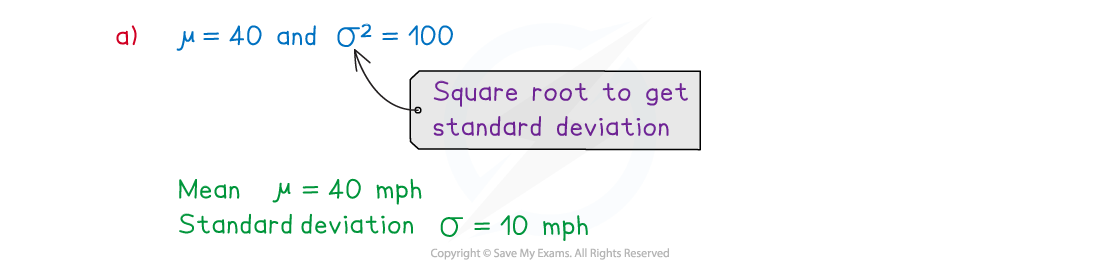(b)State the two assumptions that have been made in order to use this model.#### Exam Tip

Remember the second number in N(x, y) is the variance, if you want the standard deviation then you need to square root it.